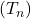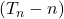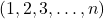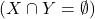How Cheenta works to ensure student success?
Explore the Back-Story

# INMO 2013 Question No. 4 Solution4     Let N be an integer greater than 1 and letbe the number of non empty subsets S of ({1,2,.....,n}) with the property that the average of the elements of S is an integer.Prove thatis always even.

Sketch of the Proof:= number of nonempty subsets ofwhose average is an integer. Call these subsets int-avg subset (just a name)

Note that one element subsets are by default int-avg subsets. They are n in number. Removing those elements fromwe are left with int-avg subsets with two or more element. We want to show that the number of such subsets is even.

Let X be the collection of all int-avg subsets S such that the average of S is contained in S
Y be the set of all int-avg subsets S such that the average of S is not contained in S.

Adding or deleting the average of a set to or from that set does not change the average.
This operation sets up a one-to-one correspondence between X and Y, so X and Y have the same cardinality. Since, the number of elements inis even and hence the number of subsets of two or more elements that have an integer average is even.

Comment

What is the cardinality of?

4     Let N be an integer greater than 1 and letbe the number of non empty subsets S of ({1,2,.....,n}) with the property that the average of the elements of S is an integer.Prove thatis always even.

Sketch of the Proof:= number of nonempty subsets ofwhose average is an integer. Call these subsets int-avg subset (just a name)

Note that one element subsets are by default int-avg subsets. They are n in number. Removing those elements fromwe are left with int-avg subsets with two or more element. We want to show that the number of such subsets is even.

Let X be the collection of all int-avg subsets S such that the average of S is contained in S
Y be the set of all int-avg subsets S such that the average of S is not contained in S.

Adding or deleting the average of a set to or from that set does not change the average.
This operation sets up a one-to-one correspondence between X and Y, so X and Y have the same cardinality. Since, the number of elements inis even and hence the number of subsets of two or more elements that have an integer average is even.

Comment

What is the cardinality of?

This site uses Akismet to reduce spam. Learn how your comment data is processed.

### One comment on “INMO 2013 Question No. 4 Solution”

1.Rahul says:

T_n - n is the number of non-singleton sets ......ar non- singleton sets always occur in pairs,er modhye there exists a S which contains the average.amr mne hoy this is a shorter solution.i was working it out.....

### Knowledge Partner